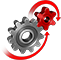# WolframSystemModeler

## Optimal Control for a Continuously Stirred Tank Reactor

Find an optimal control signal for a continuously stirred tank reactor (CSTR). The temperature in the tank influences a reaction rate, while the temperature in the cooling jacket acts as a control signal. The goal is to go from one concentration to another as quickly as possible while obeying safety limitations in temperature.

To run this example, you'll need

Analyze the System

Get the equations governing tank behavior into Mathematica for in-depth analysis.

Interactively Study Model Behavior

Adjust the control signal interactively and instantly see how the system responds.Concentration, tank temperature, and cooling
temperature from red starting level to green target level
Dashed line shows optimal control result.

Optimize Control Signals with Mathematica

Use the optimization functionality in Mathematica to find an optimal cooling temperature.

## Wolfram System Modeler

Questions? Comments? Contact a Wolfram expert »